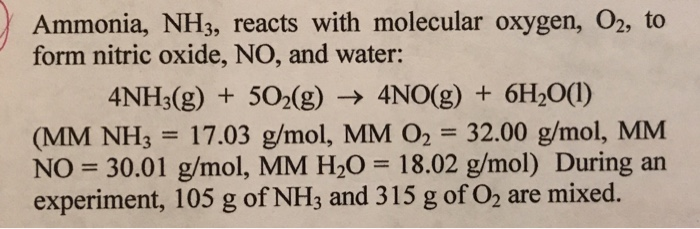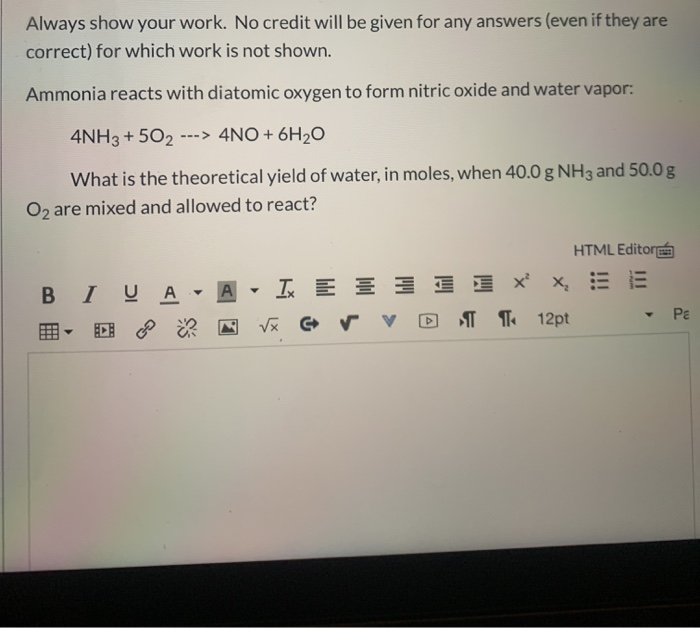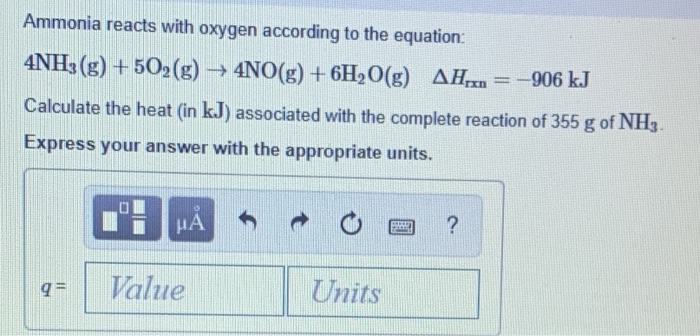Question

# Ammonia reacts with oxygen to form nitric oxide and water vapor: 4NH3 +5O2 → 4NO + 6H2O

Ammonia reacts with oxygen to form nitric oxide and water vapor:

4NH3 +5O2 → 4NO + 6H2

What is the theoretical yield of water, in moles, when 40.0g NH3 and 50.0g O2 are mixed and allowed to react:

•  1.87 mol

•  3.53 mol

•  1.57 mol

•  1.30 mol

•  None of these

Number of moles of NH3= mass/mol. wt .=40.0 g/ (17.03 g/mol)=2.348796 mol

Number of moles of O2= mass / mol . wt .=50.0 g /(17.03 g / mol)=1.5625 mol

According to balanced equation,

5 moles of O2 reacts with 4 moles of NH3

So, 1.5625 mol of O2 would react with 1.5625 mol * 4 / 5 = 1.25 mol of NH3

But the number of moles of NH3 present is more than required.

That is NH3 is the excess reagent.

O2 is the Limiting reagent.

According to the balanced equation,

5 moles of O2 produces 6 moles of H2O

So, 1.5625 mol of O2 would produce 1.5625 mol * 6 / 5=1.875 mol of H2O

The number of moles of H2O = 1.87 mol

Option A is the correct answer.

#### Earn Coins

Coins can be redeemed for fabulous gifts.

Similar Homework Help Questions
• ### Ammonia reacts with oxygen to form nitric oxide and water vapor: 4NH3 + 5O2 ---> 4NO...

Ammonia reacts with oxygen to form nitric oxide and water vapor: 4NH3 + 5O2 ---> 4NO + 6H2O When 20.0 g NH3 and 50.0 g O2 are allowed to react, which is the limiting reagent? A) NO B) O2 C) H2O D) no reagent is limiting E) NH3 What is the coefficient for O2 when the following combustion reaction of a hydrocarbon is balanced? ___C7H14 + ___ O2 ---> ___ CO2 + __ H2O A) none of these B) 10...

• ### 1) Ammonia, NH3, reacts with molecular oxygen, O2, to formnitric oxide, NO, and water:4NH3(g)...1) Ammonia, NH3, reacts with molecular oxygen, O2, to form nitric oxide, NO, and water:4NH3(g) + 5O2(g) = 4NO (g) +6H2O(l)A. What is the limiting reactant and what is the theoretical yield of NO?B. What is the theoretical yield of H2O?C. How many grams of excess reagent will be left over?D. If the actual yield of NO had been 91 g, what would be the percent yield of the reaction

• ### Always show your work. No credit will be given for any answers (even if they are...Always show your work. No credit will be given for any answers (even if they are correct) for which work is not shown. Ammonia reacts with diatomic oxygen to form nitric oxide and water vapor: 4NH3 + 502 ---> 4NO + 6H2O What is the theoretical yield of water, in moles, when 40.0 g NH3 and 50.0g, O2 are mixed and allowed to react? HTML Editore BIVA - A - Ix E 1 1 1 1 XX, DE - D...

• ### Always show your work. No credit will be given for any answers (even if they are...Always show your work. No credit will be given for any answers (even if they are correct) for which work is not shown. Ammonia reacts with diatomic oxygen to form nitric oxide and water vapor: 4NH3 + 502 ---> 4NO + 6H2O What is the theoretical yield of water, in moles, when 40.0 g NH3 and 50.0g, O2 are mixed and allowed to react? HTML Editore BIVA - A - Ix E 1 1 1 1 XX, DE - D...

• ### In the first step of the Ostwald process for the synthesis of nitric acid, ammonia is converted to nitric oxide by the high-temperature reaction:4NH3(g)+5O2(g)+6H2O(g)

In the first step of the Ostwald process for the synthesis of nitric acid, ammonia is converted to nitric oxide by the high-temperature reaction:4NH3(g)+5O2(g)+6H2O(g). How is the rate of consumption of O2 related to the rate of consumption of NH3?

• ### 1. Ammonia reacts with oxygen according to the equation 4NH3(g)+5O2(g)→4NO(g)+6H2O(g),ΔHrxn=−906 kJ Calculate the heat...

1. Ammonia reacts with oxygen according to the equation 4NH3(g)+5O2(g)→4NO(g)+6H2O(g),ΔHrxn=−906 kJ Calculate the heat (in kJ) associated with the complete reaction of 155 g of NH3. 2. What mass of butane in grams is necessary to produce 1.5×103 kJ of heat? What mass of CO2 is produced? Assume the reaction to be as follows: C4H10(g)+132O2(g)→4CO2(g)+5H2O(g),ΔHrxn=−2658 kJ

• ### Part A Ammonia reacts with oxygen according to the equation 4NH3(g)+5O2(g)→4NO(g)+6H2O(g),ΔHrxn=−906 kJ Calculate the h...

Part A Ammonia reacts with oxygen according to the equation 4NH3(g)+5O2(g)→4NO(g)+6H2O(g),ΔHrxn=−906 kJ Calculate the heat (in kJ) associated with the complete reaction of 155 g of NH3. Part B What mass of butane in grams is necessary to produce 1.5×103 kJ of heat? What mass of CO2 is produced? Assume the reaction to be as follows: C4H10(g)+132O2(g)→4CO2(g)+5H2O(g),ΔHrxn=−2658 kJ

• ### The first step in the Oswald process for producing nitric acid is 4NH+5o2- 4NO + 6H2O...

The first step in the Oswald process for producing nitric acid is 4NH+5o2- 4NO + 6H2O if 132g of ammonia reacts with 132g of oxygen A) how many grams of nk itric oxide(No) will be produced B) which compound is limiting reagent C) what is the % yield of this reaction if actual yield of NO is 67.Og?

• ### Equation: 4NH3(g) + 5O2(g) -> 4NO(g) + 6H2O(g) question: What volume of NH3 is needed to react with 71.6 liters of oxygen

Equation: 4NH3(g) + 5O2(g) -> 4NO(g) + 6H2O(g) question: What volume of NH3 is needed to react with 71.6 liters of oxygen?

• ### Ammonia reacts with oxygen according to the equation: 4NH3(g) +502(g) → 4NO(g) + 6H2O(g) AH.,.. =...Ammonia reacts with oxygen according to the equation: 4NH3(g) +502(g) → 4NO(g) + 6H2O(g) AH.,.. = 4906 kJ Calculate the heat (in kJ) associated with the complete reaction of 355 g of NH3. Express your answer with the appropriate units. Value Units